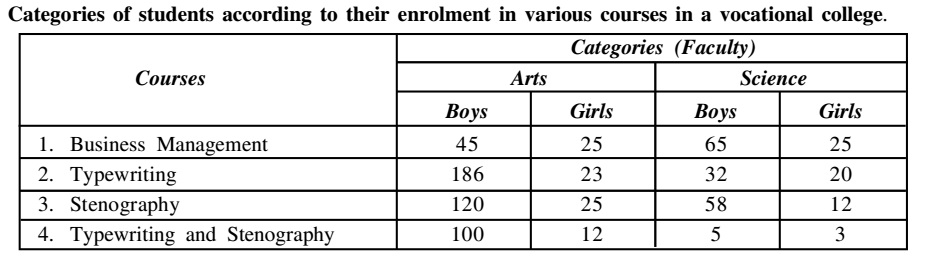## Table chart

#### Data Interpretation

Direction: Study the following table and answer the question given below:1. If 60% boys and 70% girls pass in their respective courses, then total pass percentage is :

1. ∵ Total boys = 45 + 186 +120 +100 + 65 + 32 + 58 + 5 = 611

Total number of girls = 25 + 23 + 25 + 12 + 25 + 20 + 12 + 3 = 145

##### Correct Option: D

∵ Total boys = 45 + 186 +120 +100 + 65 + 32 + 58 + 5 = 611
∴ Number of boys who pass in their respective courses = 60% of 611 = 611 x 60/100 = 366.60
Total number of girls = 25 + 23 + 25 + 12 + 25 + 20 + 12 + 3 = 145
Number of girls who pass in their respective courses =70% of 145= 145 x 70/100 = 101.50
∴ Combined pass percent = (366.6 + 101.5) x 100/(611 + 145) = 46810/756 = 61.92 = 62%

1. Total number of boys studying in the college exceeds the total number of girls in the college by:

1. Total number of boys = 45 + 65 + 186 + 32 + 120 + 58 + 100 + 5 = 611
Total number of girls = 25 + 23 + 25 + 12 + 25 + 20 + 12 + 3 = 145

##### Correct Option: A

Total number of boys = 45 + 65 + 186 + 32 + 120 + 58 + 100 + 5 = 611
Total number of girls = 25 + 23 + 25 + 12 + 25 + 20 + 12 + 3 = 145
∴ Required percentage = (611 - 145/145) x 100 ≈ 321%

1. In the art faculty number of students who have opted the course of stenography is how much percent of the total student in the faculty?

1. Required percentage = (25 + 120) x 100/536

##### Correct Option: A

Required percentage = (25 + 120) x 100/536 = 100≈ 27

1. The total number of students who have opted the course of Business Management and the course of Stenography exceeds the number of students studying in typewriting course by:

1. ∵ Number of students in Business Management course = 160
Number of students in typewriting course = 261
Number of students in stenography course = 215

##### Correct Option: A

∵ Number of students in Business Management course = 160
Number of students in typewriting corse = 261
Number of students in stenography corse = 215
∴ Requied percent = (160 + 215 - 261/261) x 100 ≈ 44%

1. In which of the following courses, percentage of girls is the highest?

1. Percentage of girls in Business Management course = (25 + 25) x 100/160 = 31.25
Percentage of girls in typewriting course = (23 + 20) x 100/261 = 16.47
Percentage of girls in stenography course = (25 + 12) x 100/215 =12.56
And percentage of girls in typewriting and stenography course = (12 + 3) x 100/120 = 12.50

##### Correct Option: B

Percentage of girls in Business Management course = (25 + 25) x 100/160 = 31.25
Percentage of girls in typewriting course = (23 + 20) x 100/261 = 16.47
Percentage of girls in stenography course = (25 + 12) x 100/215 =12.56
And percentage of girls in typewriting and stenography course = (12 + 3) x 100/120 = 12.50
Hence, it is clear from the above that percentage of girls in Business Management course is the highest,